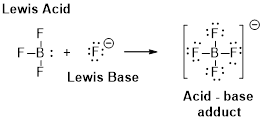# Decide whether each of the following substances should be classified as a Lewis acid or a Lewis base. (a) H 2 NOH in the reaction H 2 NOH(aq)+HCl(aq) → [H 3 NOH]Cl(aq) (b) Fe 2+ (c) CH 3 NH 2 ( Hint : Draw the electron dot structure)### Chemistry & Chemical Reactivity

9th Edition
John C. Kotz + 3 others
Publisher: Cengage Learning
ISBN: 9781133949640

#### Solutions

Chapter
Section### Chemistry & Chemical Reactivity

9th Edition
John C. Kotz + 3 others
Publisher: Cengage Learning
ISBN: 9781133949640
Chapter 16, Problem 79PS
Textbook Problem
9 views

## Decide whether each of the following substances should be classified as a Lewis acid or a Lewis base. (a) H 2 NOH in the reaction H 2 NOH(aq)+HCl(aq)  →  [H 3 NOH]Cl(aq) (b) Fe 2+ (c) CH 3 NH 2 (Hint: Draw the electron dot structure)

(a)

Interpretation Introduction

Interpretation:

H2NOH Whether a Lewis acid or a Lewis base has to be identified.

Concept Introduction:

Lewis definition:

A Lewis acid is a substance that can accept and share an electron pair.

A Lewis base is a substance that can donate and share an electron pair.### Explanation of Solution

The structure of H2NOH, hydroxyl amine is as follows.

(b)

Interpretation Introduction

Interpretation:

Fe2+ Whether a Lewis acid or a Lewis base has to be identified.

Concept Introduction:

Lewis definition:

A Lewis acid is a substance that can accept and share an electron pair.

A Lewis base is a substance that can donate and share an electron pair.(c)

Interpretation Introduction

Interpretation:

CH3NH2 Whether a Lewis acid or a Lewis base has to be identified.

Concept Introduction:

Lewis definition:

A Lewis acid is a substance that can accept and share an electron pair.

A Lewis base is a substance that can donate and share an electron pair.### Still sussing out bartleby?

Check out a sample textbook solution.

See a sample solution

#### The Solution to Your Study Problems

Bartleby provides explanations to thousands of textbook problems written by our experts, many with advanced degrees!

Get Started

Find more solutions based on key concepts
The body's adipose tissue has a virtually infinite capacity to store fats. T F

Nutrition: Concepts and Controversies - Standalone book (MindTap Course List)

List the differences between mitosis and meiosis in the following chart:

Human Heredity: Principles and Issues (MindTap Course List)

How does a muscle fiber incur an oxygen debt?

Human Biology (MindTap Course List)

In what part of the cell does glycolysis take place?

Chemistry for Today: General, Organic, and Biochemistry

Match the term listed in Column A with its definition from Column B

Nutrition Through the Life Cycle (MindTap Course List)

Determine the initial direction of the deflection of charged particles as they enter the magnetic fields shown ...

Physics for Scientists and Engineers, Technology Update (No access codes included)

Is the greenhouse effect always a bad thing?

Oceanography: An Invitation To Marine Science, Loose-leaf Versin